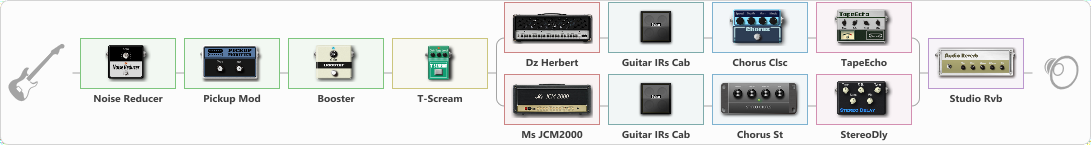# Darkness

Discussion in 'ToneLib-GFX presets' started by truss van halen, Dec 10, 2020.

1. ### truss van halenWell-Known Member

Darkness

Preset name: Darkness

Effects chain:Effect: "Noise Reducer" (Dynamics / Filter), active - "yes"
{
"Sens" = 89
"Mode" = Hard
}

Effect: "Pickup Mod" (Dynamics / Filter), active - "yes"
{
"Mode" = Phase Out
"Tone" = 50
}

Effect: "Booster" (Dynamics / Filter), active - "yes"
{
"Gain" = 25
}

Effect: "T-Scream" (Overdrive / Distortion), active - "yes"
{
"Drive" = 9
"Tone" = 80
"Level" = 79
}

Effect: "Splitter" (Dynamics / Filter), active - "yes"
{
"A-Bypass" = Off
"A-Pan" = 0
"A-Level" = 55
"B-Bypass" = Off
"B-Pan" = 0
"B-Level" = 55

'A' branch:
{

Effect: "Dz Herbert" (Amp simulators), active - "yes"
{
"Gain" = 51
"Bass" = 62
"Middle" = 63
"Treble" = 66
"Presence" = 51
"Master" = 70
"Level (dB)" = 2
}

Effect: "Guitar IRs Cab" (Cabinets), active - "yes"
{
"Model" = Marshall JCM2000 (4x12")
"Mic Position" = Middle
"Mic Distance" = Middle
"Low Cut (Hz)" = 60
"Hi Cut (kHz)" = 20.0
"Mix" = 100
"Level (dB)" = 0
}

Effect: "Chorus Clsc" (Modulation / Sfx), active - "yes"
{
"Speed" = 3.5
"Depth" = 58
"Center" = 1.0
"Mode" = Stereo
}

Effect: "TapeEcho" (Delay), active - "yes"
{
"Time" = 316
"Feedback" = 9
"Tone" = 65
"LoDamp" = 19
"Mix" = 53
}
}
'B' branch:
{

Effect: "Ms JCM2000" (Amp simulators), active - "yes"
{
"Gain" = 51
"Bass" = 48
"Middle" = 51
"Treble" = 63
"Presence" = 63
"Master" = 70
"Level (dB)" = 2
}

Effect: "Guitar IRs Cab" (Cabinets), active - "yes"
{
"Model" = Marshall 1960b (4x12")
"Mic Position" = Middle
"Mic Distance" = Near
"Low Cut (Hz)" = 60
"Hi Cut (kHz)" = 20.0
"Mix" = 100
"Level (dB)" = 0
}

Effect: "Chorus St" (Modulation / Sfx), active - "yes"
{
"Speed" = 1.9
"Depth" = 31
"Center" = 4.4
"Mix" = 40
}

Effect: "StereoDly" (Delay), active - "yes"
{
"Time" = 756
"Feedback" = 14
"Tone" = 76
"Sens" = 32
"Mix" = 46
}
}
}

Effect: "Studio Rvb" (Reverberation), active - "yes"
{
"Time" = 7.3
"PreDelay" = 48
"LoDamp" = 21
"HiDamp" = 23
"Mix" = 30
}

Note: You will need to download and install the ToneLib-GFX software to use the preset.

#### Attached Files:

• ###### Darkness.tlgfx
File size:
1.2 KB
Views:
2,881
Skeve Nelis, Randall, SEMENOV and 8 others like this.